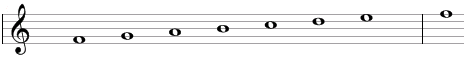# Lydian scale

The Lydian scale is a primary heptatonic scale that can be built using the following steps between notes: 1, 1, 1, ½, 1, 1, ½.

One example of this scale is F, G, A, B, C, D, E. This example is shown below in traditional notation and in guitar tablature notation.The Lydian scale is the fourth mode of standard major scale or the sixth mode of the natural minor scale.

The Lydian scale is a "primary" heptatonic scale (heptonia prima) as it uses only either one or two semitone intervals between any two adjacent notes and as the two single-semitone intervals are spaced as evenly as possible. If we start from the interval of one semitone between B and C we will get two intervals of two semitones – between C and D and D and E – and get to the second interval of one semitone – between E and F. If go in the opposite direction, we will go through three intervals of two semitones – between A and B, G and A, and F and G.

## Modes of the Lydian scale

The following are modes of the Lydian scale.

1. Lydian, with steps 1, 1, 1, ½, 1, 1, ½ (F, G, A, B, C, D, E in the example above).
2. Mixolydian, with steps 1, 1, ½, 1, 1, ½, 1 (G, A, B, C, D, E, F).
3. Aeolian or natural minor, with steps 1, ½, 1, 1, ½, 1, 1 (A, B, C, D, E, F, G).
4. Locrian, with steps ½, 1, 1, ½, 1, 1, 1 (B, C, D, E, F, G, A).
5. Ionian or major, with steps 1, 1, ½, 1, 1, 1, ½ (C, D, E, F, G, A, B).
6. Dorian, with steps 1, ½, 1, 1, 1, ½, 1 (D, E, F, G, A, B, C).
7. Phrygian, with steps ½, 1, 1, 1, ½, 1, 1 (E, F, G, A, B, C, D).

## Three-note chords on the Lydian scale

The following are common triads built on the notes of the Lydian scale.

• On the root of the scale (on the tonic): major chord (e.g., F composed of F, A, C) or suspended chord (Fsus2 = F, G, C).
• On the second note (on the supertonic): major chord (G = G, B, D) or suspended chords (Gsus4 = G, C, D and Gsus2 = G, A, D).
• On the third note (on the mediant): minor chord (Am = A, C, E) or suspended chords (Asus4 = A, D, E and Asus2 = A, B, E).
• On the fourth note (on the subdominant): diminished chord (Bdim = B, D, F).
• On the fifth note (on the dominant): major chord (C = C, E, G) or suspended chords (Csus4 = C, F, G and Csus2 = C, D, G).
• On the sixth note (on the submediant): minor chord (Dm = D, F, A) or suspended chords (Dsus4 = D, G, A and Dsus2 = D, E, A).
• On the seventh note (on the leading tone): minor chord (Em = E, G, B) or suspended chord (Esus4 = E, A, B).

## Four-note chords on the Lydian scale

The following are seventh chords built on the notes of the Lydian scale.

• On the first note: major seventh chord (e.g., Fmaj7 composed of F, A, C, E).
• On the second note: dominant seventh chord (G7 = G, B, D, F).
• On the third note: minor seventh chord (Amin7 = A, C, E, G).
• On the fourth note: half-diminished seventh chord (minor seventh chord with a flat fifth, Bm7b5 = B, D, F, A).
• On the fifth note: major seventh chord (Cmaj7 = C, E, G, B).
• On the sixth note: minor seventh chord (Dmin7 = D, F, A, C).
• On the seventh note: minor seventh chord (Emin7 = E, G, B, D).

## Intervals on the Lydian scale

The Lydian scale is composed of the following intervals.

• A major second, e.g., the interval between F and G is equal to two semitones.
• A major third, the interval between F and A is equal to four semitones.
• An augmented fourth, the interval between F and B is equal to six semitones.
• A perfect fifth, the interval between F and C is equal to seven semitones.
• A major sixth, the interval between F and D is equal to nine semitones.
• A major seventh, the interval between F and E is equal to eleven semitones.

Scale, Scale (index)

### Filtered HTML

• Freelinking helps you easily create HTML links. Links take the form of [[indicator:target|Title]]. By default (no indicator): Click to view a local node.